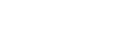## User Counts of ratio of answer to question

0

(click on this box to dismiss)Q&A for Ubuntu users and developers

``````SELECT
COUNT(pvt.owneruserid) UserCount,
ROUND(CASE
WHEN pvt.Question <> 0 THEN

/
cast(pvt.Question as decimal(7,2))

END, 2) ratio

FROM   (SELECT p.owneruserid,
p.id,
pt.name
FROM   posts p
INNER JOIN posttypes pt
ON p.posttypeid = pt.id
WHERE pt.ID in (1,2) and p.ownerUserID is not null) p
PIVOT (
COUNT (p.id)
FOR p.name IN ([Question], [Answer])) AS pvt
GROUP BY
ROUND(CASE
WHEN pvt.Question <> 0 THEN

/
cast(pvt.Question as decimal(7,2))

END, 2)
ORDER BY
ROUND(CASE
WHEN pvt.Question <> 0 THENHold tight while we fetch your results